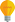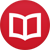# Explanation of integration by parts

by Jakub MarianTip: See my list of theMost Common Mistakes in English. It will teach you how to avoid mis­takes with com­mas, pre­pos­i­tions, ir­reg­u­lar verbs, and much more.

Integration by parts is one of the first methods people learn in calculus courses. Together with integration by substitution, it will allow you to solve most of the integrals students get in exams and tests. The usual formulation goes as follows:

$$∫ u’(x)v(x)\,dx = u(x)v(x) - ∫ u(x)v’(x)\,dx$$

That is, whenever you have an expression of the form $u’(x)v(x)$ inside an integral, you can rewrite the whole integral as the right-hand side above. Why is this true? The reason is actually very simple. First, start with the product rule for derivatives:

$$\l(u(x)v(x)\r)’ = u’(x)v(x) + u(x)v’(x)$$

Move the second term on the right-hand side to the left and flip the equation:

$$u’(x)v(x) = \l(u(x)v(x)\r)’ - u(x)v’(x)$$

Now, integrate both sides. The derivative cancels with the integral, i.e. $∫ \l(u(x)v(x)\r)’\,dx = u(x)v(x) + c$, and we get the formula given at the beginning of this article (note that we don’t have to write “$+\,c$” there because we will always add it when we solve the remaining integral).

A better way to apply the formula in practice is to think of the following pattern

$$∫ fg = Fg - ∫ Fg’$$

where $F$ is the integral of $f$ (or, more precisely, any primitive function of $f$). When you have a product, you decide which function to integrate ($f$) and which to differentiate ($g$); then you have only the integrated function ($F$) on the right-hand side, first with the original $g$ and then with the differentiated $g’$.

## Examples

Let’s solve a couple of simple integrals:

$$∫ \overbrace{x\vphantom{e^x}}^g\overbrace{e^x}^f\,dx = \overbrace{x\vphantom{e^x}}^g\overbrace{e^x}^F - ∫ \overbrace{1}^{g’}\overbrace{e^x}^F\,dx = xe^x - e^x +c = e^x(x-1) + c$$

What happened there? We decided that we should integrate $e^x$ and differentiate $x$ (usually you want to integrate something that does not become more complicated and differentiate something that becomes simpler).

Here’s another example of the same principle:

$$∫ \overbrace{x\vphantom{\cos(x)}}^g\overbrace{\cos(x)}^f\,dx = \overbrace{x\vphantom{\sin(x)}}^g\overbrace{\sin(x)}^F - ∫ \overbrace{1}^{g’}\overbrace{\sin(x)}^F\,dx = x\sin(x) + \cos(x) +c$$

These examples may lead you to believe that whenever you integrate a product of $x$ and something else, you always differentiate $x$. However, the point is that we want the differentiated part to become simpler ($x$ is simple enough already in many cases) because integration rarely leads to simplification. Take a look at the following example:

$$∫ x\ln(x)\,dx\,,$$

where $\ln(x)$ is the natural logarithm. The primitive function of $\ln(x)$ is probably not something you have memorized (and it would not help us much here anyway). On the other hand, the derivative of $\ln(x)$ is just $\frac{1}x$, which is a very simple function. Unlike the previous examples, it makes more sense to integrate $x$ and differentiate the rest here:

$$∫ \overbrace{x\vphantom{\ln(x)}}^f\overbrace{\ln(x)}^g\,dx = \overbrace{\frac{x^2}2}^F\overbrace{\ln(x)\vphantom{\frac{x^2}2}}^g - ∫ \overbrace{\frac{x^2}2}^F \overbrace{\frac{1}x}^{g’}\,dx = \frac{x^2}2\ln(x)-\frac12 ∫ x\,dx \\= \frac{x^2}2\ln(x) - \frac12 \frac{x^2}2 + c = \frac{x^2}2\l(\ln(x)-\frac12\r) + c\,.$$

Sometimes it is useful to apply integration by parts repeatedly to gradually simplify the expression we have. This often happens when there is a power of $x$ greater than one:

$$∫ \overbrace{x^2}^g\overbrace{\sin(x)}^f\,dx = \overbrace{x^2}^g\overbrace{(-\cos(x))}^F - ∫ \overbrace{2x}^{g’}\overbrace{(-\cos(x))}^F \,dx \\ = -x^2\cos(x) + 2∫x\cos(x)\,dx$$

The integral on the right-hand side can be solved via integration by parts, and, in fact, we did that as the second example in this section, so instead of solving it again, we will use the former result $∫ x\cos(x)\,dx = x\sin(x) + \cos(x) +c$:

$$∫ x^2\sin(x)\,dx = -x^2\cos(x) + 2x\sin(x)+2\cos(x)+c\,.$$

You may be wondering why I wrote $c$ instead of $2c$. This is merely a notational conventionthe final constant of integration is usually called $c$, even if there were other $c$’s in the process.

## Definite integration

The usual way to calculate $∫_a^b f(x)\,dx$ is to calculate the indefinite integral first and then apply the limits to the result, and integration by parts is no exception. For example, we could calculate $∫_0^{\pi} x\cos(x)$ using the solution above as:

$$∫_0^\pi x\cos(x)\,dx = \l[x\sin(x) + \cos(x)\r]_0^\pi\\ = \pi\sin(\pi) + \cos(\pi) - (0\sin(0) + \cos(0)) = -1 - 1 = -2.$$

(the notation $[f(x)]_{a}^b$ means $f(b)-f(a)$ or $\lim_{x→b-} f(x) - \lim_{x→a+} f(x)$ if $f(x)$ is not defined at some of the points).

However, it is sometimes useful to apply the limits in integration by parts directly, without knowing the solution. The definite version of the theorem can be written as:

$$∫_a^b u’(x)v(x)\,dx = \l[u(x)v(x)\r]_{a}^b - ∫_a^b u(x)v’(x)\,dx$$

This formula can simplify calculations because it eliminates the need to find the entire primitive function before applying the limits. For example:

$$∫_0^∞ x^3e^{-x}\,dx = \l[x^3(-e^{-x})\r]_0^∞ + ∫_0^∞ 3x^2e^{-x}\,dx \\ = 0 + \l[3x^2(-e^{-x})\r]_0^∞ + ∫_0^∞ 6xe^{-x}\,dx \\ = 0 + \l[6x(-e^{-x})\r]_0^∞ + ∫_0^∞ 6e^{-x}\,dx \\ = 0 + \l[-6e^{-x}\r]_0^∞ = 6$$

Notice that the expression in the square brackets is always $0$ (except the very last term) because $\lim_{x→∞} x^ne^{-x} = 0$, and for $x = 0$ and $n > 0$, the expression is also $0$.

What if we calculated $∫_0^∞ x^4e^{-x}$ instead? Perhaps you can see the pattern in the expressions above. In the first step we would get $4x^3$, then $4⋅3⋅x^2$, then $4⋅3⋅2⋅x$, and then $4⋅3⋅2⋅1 = 4!$. It seems that in general, $∫_0^∞ x^ne^{-x}\,dx = n!$, but how to prove it?

There is a simple proof by mathematical induction using the formula above. It is easy to calculate that the formula holds for $n = 1$. Now, suppose we know that $∫_0^∞ x^ne^{-x}\,dx = n!$ for a given $n$; all we need to do is to prove that it also holds for $n+1$:

$$∫_0^∞ x^{n+1}e^{-x}\,dx = \l[x^{n+1}(-e^{-x})\r]_0^∞ + (n+1)∫_0^∞ x^ne^{-x}\,dx \\ = 0 + (n+1)n! = (n+1)!$$

QED. We used the fact that the bracket is equal to $0$ and the assumption that $∫_0^∞ x^ne^{-x}\,dx = n!$. It would be much harder to prove this without the formula for definite integration by parts. By the way, the integral $∫_0^∞ x^{n}e^{-x}\,dx$ gives us a way to generalize factorials to non-whole numbers (because, for instance, $∫_0^∞ x^{1/2}e^{-x}\,dx$ makes perfect sense). Maybe integration by parts is not such a dull thing, after all…

By the way, I have written several educational ebooks. If you get a copy, you can learn new things and support this website at the same time—why don’t you check them out?